# Torsional & Bending Stresses in Machine Parts Notes | Study Mechanical Engineering SSC JE (Technical) - Mechanical Engineering

## Mechanical Engineering: Torsional & Bending Stresses in Machine Parts Notes | Study Mechanical Engineering SSC JE (Technical) - Mechanical Engineering

The document Torsional & Bending Stresses in Machine Parts Notes | Study Mechanical Engineering SSC JE (Technical) - Mechanical Engineering is a part of the Mechanical Engineering Course Mechanical Engineering SSC JE (Technical).
All you need of Mechanical Engineering at this link: Mechanical Engineering

TORSIONAL AND BENDING STRESS IN MACHINE PARTS

Torsional Stress

• When a machine member is subjected to two equal and opposite couples acting in parallel plane, then the machine member is said to be subjected to torsion. The stress set up by torsion is known as torsional shear stress.
• It is zero at the centroidal axis and maximum at the outer surface.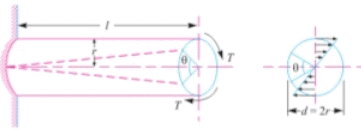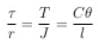where,
ζ = Torsional shear stress induced at the outer surface of the shaft.
r = Radius of the shaft.
T = Torque or twisting moment.
J = Second moment of area of the section about its polar axis.
C = Modulus of rigidity.
l = length of the shaft.
θ = Angle of twist in radians on a length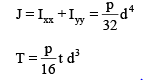Power transmitted by a shaft (in watts)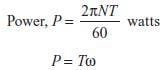N = Speed in rpm
w = Angular speed in rad/s

Bending Stress

• When a beam subjected to the bending moment, in sagging (upward bending) the fibres of the upper side of the beam will be shortened due to compression and those on the lower side will be elongated due to tension, somewhere between top and bottom fibres, there is a surface at which the fibres are neither shortened nor elongated. Such a surface is called neutral plane.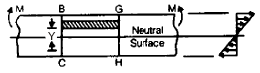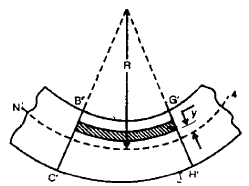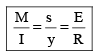where,
M = Bending moment acting at the given section
s = Bending stress
I = Moment of inertia of the cross-section about neutral axis
y = distance from the neutral axis to extreme fibre
R = Radius of curvature of beam.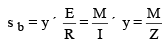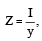is known as section modulus.

• In case of symmetrical sections such as circular, square or rectangular, the neutral axis passes through its geometrical centre.
• In case of unsymmetrical sections such as L-section or T-section the neutral axis does not pass through its geometrical centre. In such cases, all the centroid of the section is calculated and then the distance of the extreme fibres for both lower and upper side of the section is obtained. Out of these two values, the bigger value is used in bending equation.
• Principal stress,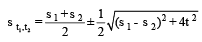•  Maximum shear stress,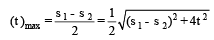• When the shaft is subjected to an axial load (P) in addition to bending and twisting moments as in the propeller shafts of ship and shafts for driving worm gears, then the resultant tensile stress or compressive stress depend upon the type of axial load.
The document Torsional & Bending Stresses in Machine Parts Notes | Study Mechanical Engineering SSC JE (Technical) - Mechanical Engineering is a part of the Mechanical Engineering Course Mechanical Engineering SSC JE (Technical).
All you need of Mechanical Engineering at this link: Mechanical EngineeringUse Code STAYHOME200 and get INR 200 additional OFF

## Mechanical Engineering SSC JE (Technical)

6 videos|89 docs|55 tests

Track your progress, build streaks, highlight & save important lessons and more!

,

,

,

,

,

,

,

,

,

,

,

,

,

,

,

,

,

,

,

,

,

;